WVGA HDMI+VGA 8bpp Graphics Driver (works with the Parallax 7" HDMI LCD) in Spin2 — Parallax Forums

# WVGA HDMI+VGA 8bpp Graphics Driver (works with the Parallax 7" HDMI LCD) in Spin2

edited 2020-12-13 22:48
I turned a little example from another thread into this graphics driver.
It's just a beginning of something but can do some things already.
It shows a splash screen and then some simple graphics.

This is in Spin2 and compiles with the Propeller Tool
(But, it's likely to be turned into FlexC shortly)

Update: I've fixed the circles and added text in the attached zip.
Update1c: Now with better line drawing routine from @dgately
Update2a: Now can do both either analog VGA or digital HDMI or both at the same time
Update3b: Now with mouse cursor and also moved basepin and cog settings to top level file. Demonstrates screen painting with mouse.

Note: You will likely have to edit the basepine settings (like "hdmi_base") in the HDMI driver (main file for Ver.3) to match the physical pins you are using for the HDMI adapter.

• All working here! ;-)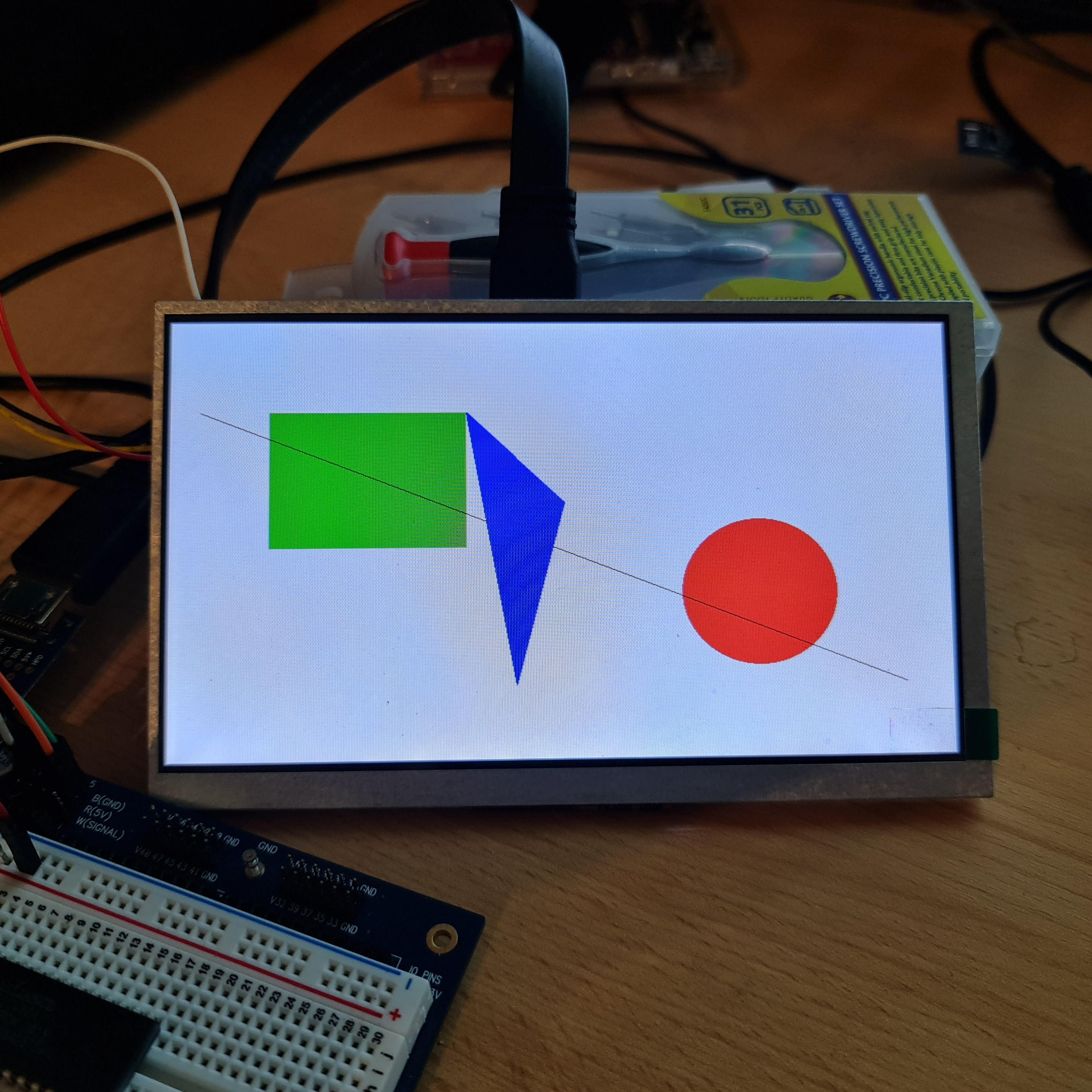• edited 2020-12-08 23:51
Ray,
Thanks for this!

Wikipedia sure helped with Bresenham's line algorithm for me (I used basically the same)

Not getting DrawCircle() to work and DrawFilledCircle() works only partially:
```vid.DrawFilledCircle(vid.Red,vid.Blue, 600,300,80)   '(c,x,y,r) only the filled part is drawn
vid.DrawCircle(vid.Black,600,300,79)   '(c,x,y,r) nothing gets drawn
```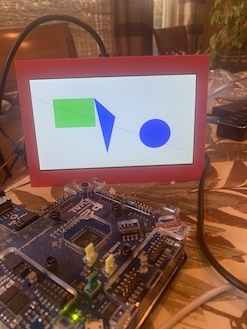I had success earlier today with this to plot a circle's circumference:
```'' From: https://web.engr.oregonstate.edu/~sllu/bcircle.pdf

x := R
y := 0
xchange := 1 - 2 * R
ychange := 1

repeat
Plot8CirclePoints(cx,cy,x,y,c)
y += 1
ychange += 2
if(2 * radiusError + xchange > 0)
x -= 1
xchange += 2
while (x >= y)

PRI Plot8CirclePoints(cx,cy,x,y,c)

SetPixel(cx+x, cy+y, c)		'' point in octant 1
SetPixel(cx-x, cy+y, c)		'' point in octant 4
SetPixel(cx-x, cy-y, c)		'' point in octant 5
SetPixel(cx+x, cy-y, c)		'' point in octant 8
SetPixel(cx+y, cy+x, c)		'' point in octant 2
SetPixel(cx-y, cy+x, c)		'' point in octant 3
SetPixel(cx-y, cy-x, c)		'' point in octant 6
SetPixel(cx+y, cy-x, c)		'' point in octant 7
```

dgately
• @Rayman Thanks for the new code. It is easier to understand because you have a separate LUT for the 24-bit colors. Given your example I'll try to put some 5x7 characters on the display. It took a while to figure out the color coding in an 8bpp bmp file and realize each bmp image has its own color table. Thank you. --Jon
• edited 2020-12-09 01:37
Thanks for testing. I've fixed the circles and added a 8x16 font.

The font looks a bit small on this screen...
• NOTE: Ensure you have the HDMI adapter connected to the P2 EVAL board's pin group that starts with 32 (32-39). @Rayman , can you note that in the top-line comments for the test program and the driver code? That info might help others get started without thinking these very-good programs don't work. Previous code had used pin group 48 (48-55). Remember, the 7-inch LCD requires an external +5V supply that can deliver at least one ampere. Thanks Ray. --Jon
• That's a good idea, I've added a note to the first post until then...
• Ray,

Possible issue with DrawLine() function...

with "PUB Main()| cz":
```repeat cz from 0 to 32 step 2
vid.DrawLine(200, 200, 200+cz * 2, 400, vid.Black)
```
When lines are drawn with X parameters close together, line does not draw as expected (if they draw at all)
DrawLine():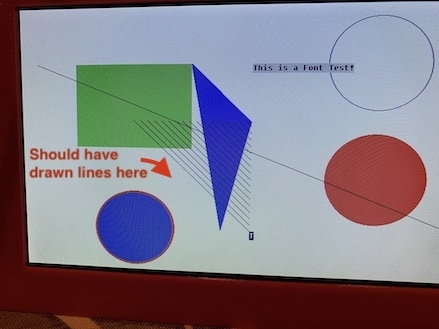PlotLine():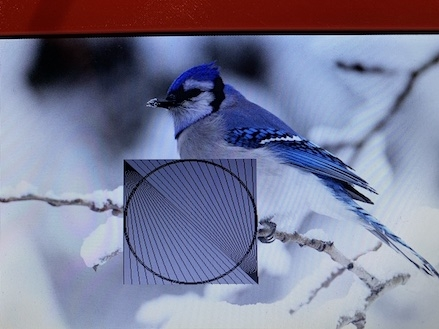My Spin2 PlotLine() code uses a pseudo-code example (from: https://en.wikipedia.org/wiki/Bresenham%27s_line_algorithm#Algorithm_for_integer_arithmetic):
```PUB plotLine(x0, y0, x1, y1,c)

if (abs(y1 - y0) < abs(x1 - x0))
if (x0 > x1)
plotLineLow(x1, y1, x0, y0,c)
else
plotLineLow(x0, y0, x1, y1,c)
else
if (y0 > y1)
plotLineHigh(x1, y1, x0, y0,c)
else
plotLineHigh(x0, y0, x1, y1,c)

PRI plotLineLow(x0, y0, x1, y1,c)| x, yi, dx, dy, D

dx := x1 - x0
dy := y1 - y0
yi := 1
if (dy < 0)
yi := -1
dy := -dy
D := 2 * dy - dx
y := y0

repeat x from x0 to x1
SetPixel(x,y,c)
if (D > 0)
y := y + yi
D := D + (2 * (dy - dx))
else
D := D + 2*dy

PRI plotLineHigh(x0, y0, x1, y1,c)| xi, x, y, dx, dy, D

dx := x1 - x0
dy := y1 - y0
xi := 1
if (dx < 0)
xi := -1
dx := -dx
D := (2 * dx) - dy
x := x0

repeat y from y0 to y1
SetPixel(x,y,c)
if D > 0
x := x + xi
D := D + (2 * (dx - dy))
else
D := D + 2*dx
```

dgately
• Excellent! I can swap yours in.
I didn’t actually test mine beyond that one line...
• @dgately Zip in top post now has your line drawing routine
• I'm looking forward to experimenting with this code some! Thanks you all for making it possible!

Doc
• Rayman wrote: »
@dgately Zip in top post now has your line drawing routine
Thx!
• I’m thinking about making this work for VGA too... Maybe either or both at same time...
• Playing with drawing rounded rectangles... Need to add filled rounded rectangles, next.

Drawing UI-type items (non-functioning):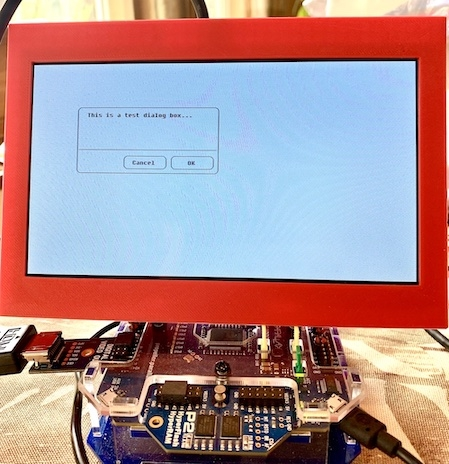Converted (to spin2) this graphic drawing code from Cornell: https://people.ece.cornell.edu/land/courses/ece4760/PIC32/TFT_display/tft_gfx.c
```PUB DrawRoundedRectangle(x,y,w,h,r,c)

'' draw sides
DrawHorizontalLine(x+r ,y ,w-2*r, c) '' top
DrawHorizontalLine(x+r ,y+h-1, w-2*r, c) '' bottom
DrawLine(x, y+r, x, y+h-r, c) '' left
DrawLine(x+w-1, y+r, x+w-1, y+h-r,c) '' right

'' draw four corners
DrawCircleHelper(x+r, y+r, r, 1, c) '' top-left
DrawCircleHelper(x+w-r-1, y+r , r, 2, c) '' top-right
DrawCircleHelper(x+w-r-1, y+h-r-1, r, 4, c) " bottom-left
DrawCircleHelper(x+r ,y+h-r-1, r, 8, c) " bottom-right

PUB DrawCircleHelper(x0, y0, r, corner, c)| f,ddF_x,ddF_y,x,y

f := 1 - r
ddF_x := 1
ddF_y := -2 * r
x := 0
y := r

repeat
if(f >= 0)
y -= 1
ddF_y += 2
f += ddF_y
x += 1
ddF_x += 2
f += ddF_x

if (corner & 4)
SetPixel(x0 + x, y0 + y, c)
SetPixel(x0 + y, y0 + x, c)
if (corner & 2)
SetPixel(x0 + x, y0 - y, c)
SetPixel(x0 + y, y0 - x, c)
if (corner & 8)
SetPixel(x0 - y, y0 + x, c)
SetPixel(x0 - x, y0 + y, c)
if (corner & 1)
SetPixel(x0 - y, y0 - x, c)
SetPixel(x0 - x, y0 - y, c)
while (x < y)
```
dgately

• edited 2020-12-11 22:51
I updated top post with a version that can also do regular VGA. Also cleaned up a bit.

I can do HDMI and analog VGA output at the same time. Or, you can pick just one.
Could probably do multiple HDMI and/or VGA at same time, but haven't tried that.

Took some work to get the VGA driver to take the basepin as a parameter. Fortunately, could steal some of that from Chip's code.
• I've updated the top post with a new version that includes mouse with cursor.
Demonstrates a Paint type app where you can draw on screen with mouse.

Basepins and cogs all in top level file now.